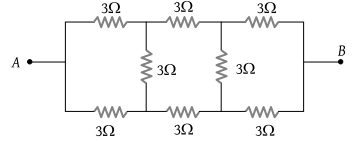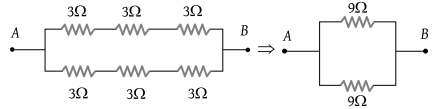Calculate the equivalent resistance between A and B(1) $\frac{9}{2}\Omega$

(2) 3 Ω

(3) 6 Ω

(4) $\frac{5}{3}\Omega$

(1) No current flow through vertical resistances${R}_{AB}=\frac{9}{2}\Omega$.

Difficulty Level:

• 63%
• 18%
• 10%
• 11%